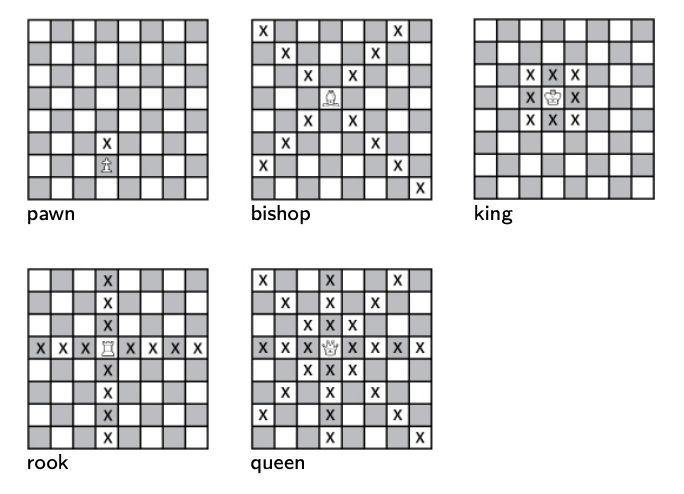시간 제한 메모리 제한 제출 정답 맞은 사람 정답 비율
2.3 초 64 MB 0 0 0 0.000%

## 문제

The mythic world of Chess Land is a rectangular grid of squares with R rows and C columns, R being greater than or equal to C. Its rows and columns are numbered from 1 to R and 1 to C, respectively.

The inhabitants of Chess Land are usually mentioned as pieces in everyday language, and there are 5 specific types of them roaming the land: pawns, rooks, bishops, queens and kings. Contrary to popular belief, chivalry is long dead in Chess Land, so there are no knights to be found.

Each piece is unique in the way it moves around from square to square: in one step,

• a pawn can move one row forward (i.e. from row r to r + 1), without changing columns;
• a rook can move any number of columns left/right without changing rows OR move any number of rows forward/backward without changing columns;
• a bishop can move to any square of the two diagonals intersecting at its currently occupied square;
• a queen can move to any square where a rook or a bishop could move to from her position;
• and a king can move to any of the 8 adjacent squares.

In the following figure, we marked by X the squares each piece can move to in a single step (here, the rows are numbered from bottom to top, and the columns from left to right).Recently, Chess Land has become a dangerous place: pieces that are passing through the land can get captured unexpectedly by unknown forces and simply disappear. As a consequence, they would like to reach their destinations as fast (i.e. in as few moves) as possible, and they are also interested in the number of different ways it is possible for them to reach it, using the minimal number of steps – because more paths being available could mean lower chances of getting captured. Two paths are considered different if they differ in at least one visited square.

For this problem, let us assume that pieces are entering Chess Land in a given column of row 1, and exit the land in a given column of row R. Your task is to answer Q questions: given the type of a piece, the column it enters row 1 and the column it must reach in row R in order to exit, compute the minimal number of moves it has to make in Chess Land, and the number of different ways it is able to do so.

## 입력

The first line contains three space separated integers R, C and Q, the number of rows and columns of Chess Land, and the number of questions, respectively. Then Q lines follow.

Each line consists of

• a character T, corresponding to the type of the piece in question (‘P’ for pawn, ‘R’ for rook, ‘B’ for bishop, ‘Q’ for queen and ‘K’ for king);
• two integers c1 and cR, 1 ≤ c1, cRC, denoting that the piece starts from the c1-th column of row 1, and has to reach the cR-th column of row R.

## 출력

You have to print Q lines, the i-th one containing two space separated integers, the answer to the i-th question: the first one is the minimal number of steps needed, the second is the number of different paths available using this number of steps. Since the answer can be quite large, you have to compute it modulo 109 + 7, using the library functions provided by the grader.

If it is impossible to reach the target square, output the line “0 0”.

## 예제 입력 1

8 8 5
P 1 2
R 4 8
Q 2 3
B 3 6
K 5 5


## 예제 출력 1

0 0
2 2
2 5
2 2
7 393